# pdeplot

Plot solution or mesh for 2-D problem

## Syntax

``pdeplot(model,"XYData",results.NodalSolution)``
``pdeplot(model,"XYData",results.Temperature,"ColorMap","hot")``
``pdeplot(model,"XYData",results.VonMisesStress,"Deformation",results.Displacement)``
``pdeplot(model,"XYData",results.ModeShapes.ux)``
``pdeplot(model,"XYData",results.ElectricPotential)``
``pdeplot(model)``
``pdeplot(mesh)``
``pdeplot(nodes,elements)``
``pdeplot(p,e,t)``
``pdeplot(___,Name,Value)``
``h = pdeplot(___)``

## Description

example

````pdeplot(model,"XYData",results.NodalSolution)` plots the solution of a `model` at nodal locations as a colored surface plot using the default `"cool"` colormap.```

example

````pdeplot(model,"XYData",results.Temperature,"ColorMap","hot")` plots the temperature at nodal locations for a 2-D thermal analysis model. This syntax creates a colored surface plot using the `"hot"` colormap.```

example

````pdeplot(model,"XYData",results.VonMisesStress,"Deformation",results.Displacement)` plots the von Mises stress and shows the deformed shape for a 2-D structural analysis model.```

example

````pdeplot(model,"XYData",results.ModeShapes.ux)` plots the `x`-component of the modal displacement for a 2-D structural modal analysis model.```

example

````pdeplot(model,"XYData",results.ElectricPotential)` plots the electric potential at nodal locations for a 2-D electrostatic analysis model.```

example

````pdeplot(model)` plots the mesh specified in `model`.```

example

````pdeplot(mesh)` plots the mesh defined as a `Mesh` property of a 2-D `model` object of type `PDEModel`.```

example

````pdeplot(nodes,elements)` plots the mesh defined by its `nodes` and `elements`.```

example

````pdeplot(p,e,t)` plots the mesh described by `p`,`e`, and `t`.```

example

````pdeplot(___,Name,Value)` plots the mesh, the data at the nodal locations, or both the mesh and the data, depending on the `Name,Value` pair arguments. Use any arguments from the previous syntaxes.Specify at least one of the `FlowData` (vector field plot), `XYData` (colored surface plot), or `ZData` (3-D height plot) name-value pairs. Otherwise, `pdeplot` plots the mesh with no data. You can combine any number of plot types.For a thermal model, you can plot temperature or gradient of temperature.For a structural model, you can plot displacement, stress, strain, and von Mises stress. In addition, you can show the deformed shape and specify the scaling factor for the deformation plot.For an electromagnetic model, you can plot electric or magnetic potentials, fields, and flux densities.```

example

````h = pdeplot(___)` returns a handle to a plot, using any of the previous syntaxes.```

## Examples

collapse all

Create a PDE model. Include the geometry of the built-in function `lshapeg`. Mesh the geometry and plot it.

```model = createpde; geometryFromEdges(model,@lshapeg); mesh = generateMesh(model); pdeplot(model)```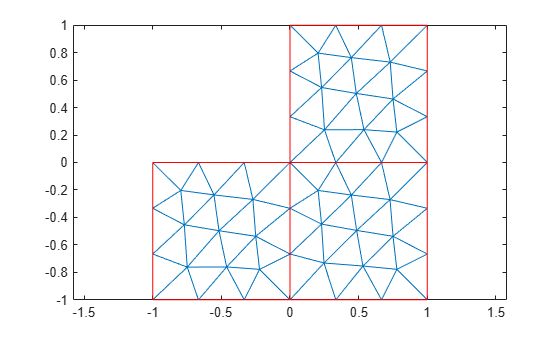Alternatively, you can plot a mesh by using `mesh` as an input argument.

`pdeplot(mesh)`Another approach is to use the nodes and elements of the mesh as input arguments for `pdeplot`.

`pdeplot(mesh.Nodes,mesh.Elements)`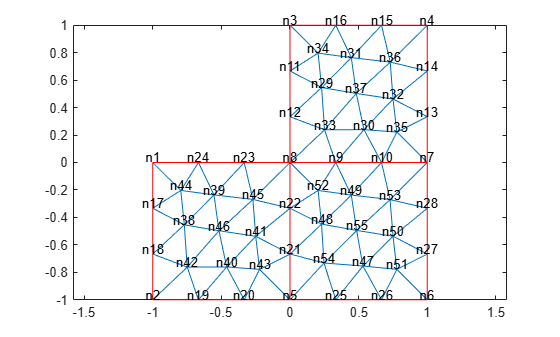Display the node labels. Use `xlim` and `ylim` to zoom in on particular nodes.

```pdeplot(model,"NodeLabels","on") xlim([-0.2,0.2]) ylim([-0.2,0.2])```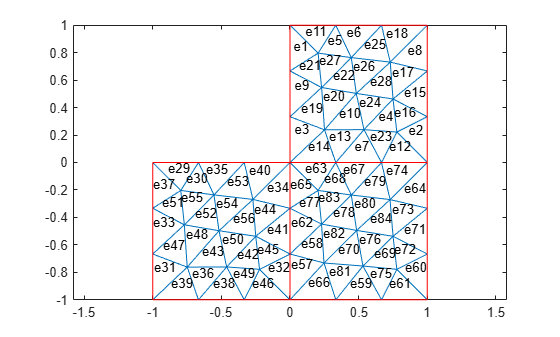Display the element labels.

```pdeplot(model,"ElementLabels","on") xlim([-0.2,0.2]) ylim([-0.2,0.2])```Create colored 2-D and 3-D plots of a solution to a PDE model.

Create a PDE model. Include the geometry of the built-in function `lshapeg`. Mesh the geometry.

```model = createpde; geometryFromEdges(model,@lshapeg); generateMesh(model);```

Set the zero Dirichlet boundary conditions on all edges.

```applyBoundaryCondition(model,"dirichlet", ... "Edge",1:model.Geometry.NumEdges, ... "u",0);```

Specify the coefficients and solve the PDE.

```specifyCoefficients(model,"m",0, ... "d",0, ... "c",1, ... "a",0, ... "f",1); results = solvepde(model)```
```results = StationaryResults with properties: NodalSolution: [1177x1 double] XGradients: [1177x1 double] YGradients: [1177x1 double] ZGradients: [] Mesh: [1x1 FEMesh] ```

Access the solution at the nodal locations.

`u = results.NodalSolution;`

Plot the 2-D solution.

`pdeplot(model,"XYData",u)`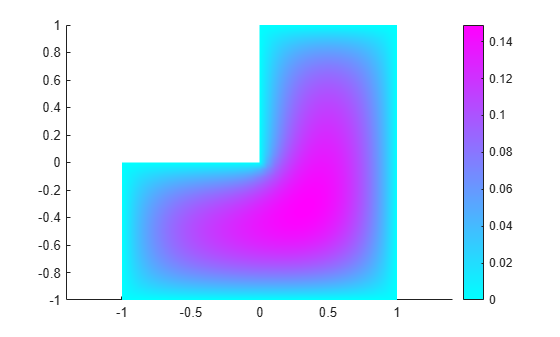Plot the 3-D solution.

`pdeplot(model,"XYData",u,"ZData",u)`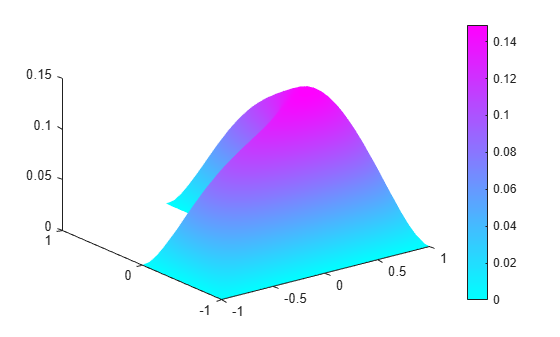Plot the gradient of a PDE solution as a quiver plot.

Create a PDE model. Include the geometry of the built-in function `lshapeg`. Mesh the geometry.

```model = createpde; geometryFromEdges(model,@lshapeg); generateMesh(model);```

Set the zero Dirichlet boundary conditions on all edges.

```applyBoundaryCondition(model,"dirichlet", ... "Edge",1:model.Geometry.NumEdges, ... "u",0);```

Specify coefficients and solve the PDE.

```specifyCoefficients(model,"m",0, ... "d",0, ... "c",1, ... "a",0, ... "f",1); results = solvepde(model)```
```results = StationaryResults with properties: NodalSolution: [1177x1 double] XGradients: [1177x1 double] YGradients: [1177x1 double] ZGradients: [] Mesh: [1x1 FEMesh] ```

Access the gradient of the solution at the nodal locations.

```ux = results.XGradients; uy = results.YGradients;```

Plot the gradient as a quiver plot.

`pdeplot(model,"FlowData",[ux,uy])`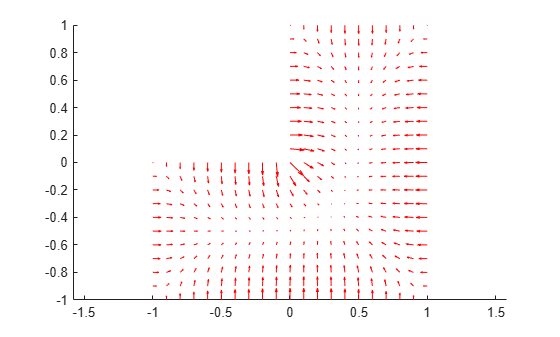Plot the solution of a 2-D PDE in 3-D with the `"jet"` coloring and a mesh, and include a quiver plot. Get handles to the axes objects.

Create a PDE model. Include the geometry of the built-in function `lshapeg`. Mesh the geometry.

```model = createpde; geometryFromEdges(model,@lshapeg); generateMesh(model);```

Set zero Dirichlet boundary conditions on all edges.

```applyBoundaryCondition(model,"dirichlet", ... "Edge",1:model.Geometry.NumEdges, ... "u",0);```

Specify coefficients and solve the PDE.

```specifyCoefficients(model,"m",0, ... "d",0, ... "c",1, ... "a",0, ... "f",1); results = solvepde(model)```
```results = StationaryResults with properties: NodalSolution: [1177x1 double] XGradients: [1177x1 double] YGradients: [1177x1 double] ZGradients: [] Mesh: [1x1 FEMesh] ```

Access the solution and its gradient at the nodal locations.

```u = results.NodalSolution; ux = results.XGradients; uy = results.YGradients;```

Plot the solution in 3-D with the `"jet"` coloring and a mesh, and include the gradient as a quiver plot.

```h = pdeplot(model,"XYData",u,"ZData",u, ... "FaceAlpha",0.5, ... "FlowData",[ux,uy], ... "ColorMap","jet", ... "Mesh","on");```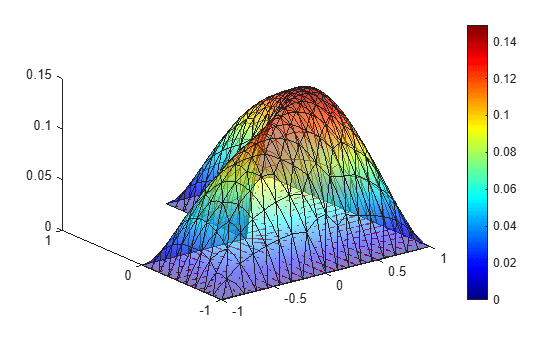Solve a 2-D transient thermal problem.

Create a transient thermal model for this problem.

`thermalmodel = createpde("thermal","transient");`

Create the geometry and include it in the model.

```SQ1 = [3; 4; 0; 3; 3; 0; 0; 0; 3; 3]; D1 = [2; 4; 0.5; 1.5; 2.5; 1.5; 1.5; 0.5; 1.5; 2.5]; gd = [SQ1 D1]; sf = 'SQ1+D1'; ns = char('SQ1','D1'); ns = ns'; dl = decsg(gd,sf,ns); geometryFromEdges(thermalmodel,dl); pdegplot(thermalmodel,"EdgeLabels","on","FaceLabels","on") xlim([-1.5 4.5]) ylim([-0.5 3.5]) axis equal```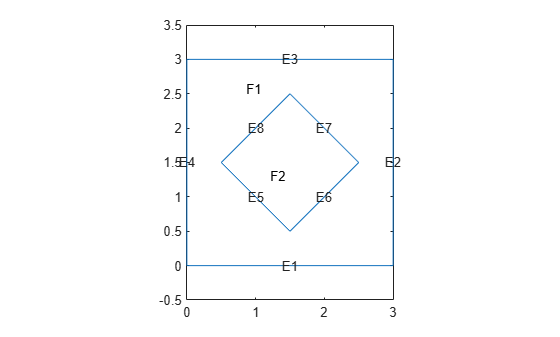For the square region, assign these thermal properties:

• Thermal conductivity is $10\text{\hspace{0.17em}}\mathrm{W}/\left(\mathrm{m}{\cdot }^{\circ }\mathrm{C}\right)$

• Mass density is $2\text{\hspace{0.17em}}\mathrm{kg}/{\mathrm{m}}^{3}$

• Specific heat is $0.1\text{\hspace{0.17em}}\mathrm{J}/\left({\mathrm{kg}\cdot }^{\circ }\mathrm{C}\right)$

```thermalProperties(thermalmodel,"ThermalConductivity",10, ... "MassDensity",2, ... "SpecificHeat",0.1, ... "Face",1);```

For the diamond region, assign these thermal properties:

• Thermal conductivity is $2\text{\hspace{0.17em}}\mathrm{W}/\left(\mathrm{m}{\cdot }^{\circ }\mathrm{C}\right)$

• Mass density is $1\text{\hspace{0.17em}}\mathrm{kg}/{\mathrm{m}}^{3}$

• Specific heat is $0.1\text{\hspace{0.17em}}\mathrm{J}/\left({\mathrm{kg}\cdot }^{\circ }\mathrm{C}\right)$

```thermalProperties(thermalmodel,"ThermalConductivity",2, ... "MassDensity",1, ... "SpecificHeat",0.1, ... "Face",2);```

Assume that the diamond-shaped region is a heat source with a density of $4\text{\hspace{0.17em}}\mathrm{W}/{\mathrm{m}}^{2}$.

`internalHeatSource(thermalmodel,4,"Face",2);`

Apply a constant temperature of 0 °C to the sides of the square plate.

`thermalBC(thermalmodel,"Temperature",0,"Edge",[1 2 7 8]);`

Set the initial temperature to 0 °C.

`thermalIC(thermalmodel,0);`

Generate the mesh.

`generateMesh(thermalmodel);`

The dynamics for this problem are very fast. The temperature reaches a steady state in about 0.1 second. To capture the most active part of the dynamics, set the solution time to `logspace(-2,-1,10)`. This command returns 10 logarithmically spaced solution times between 0.01 and 0.1.

`tlist = logspace(-2,-1,10);`

Solve the equation.

`thermalresults = solve(thermalmodel,tlist);`

Plot the solution with isothermal lines by using a contour plot.

```T = thermalresults.Temperature; pdeplot(thermalmodel,"XYData",T(:,10),"Contour","on","ColorMap","hot")```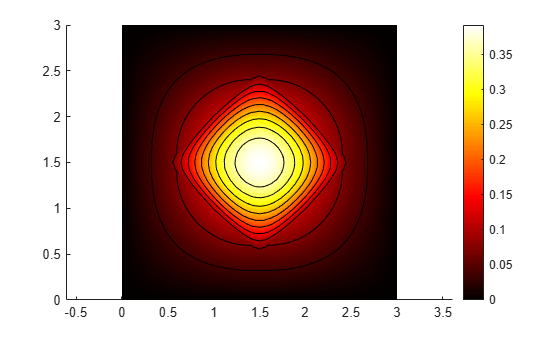Create a structural analysis model for a static plane-strain problem.

`structuralmodel = createpde("structural","static-planestrain");`

Create the geometry and include it in the model. Plot the geometry.

```geometryFromEdges(structuralmodel,@squareg); pdegplot(structuralmodel,"EdgeLabels","on") axis equal```Specify Young's modulus and Poisson's ratio.

```structuralProperties(structuralmodel,"PoissonsRatio",0.3, ... "YoungsModulus",210E3);```

Specify the x-component of the enforced displacement for edge 1.

`structuralBC(structuralmodel,"XDisplacement",0.001,"Edge",1);`

Specify that edge 3 is a fixed boundary.

`structuralBC(structuralmodel,"Constraint","fixed","Edge",3);`

Generate a mesh and solve the problem.

```generateMesh(structuralmodel); structuralresults = solve(structuralmodel);```

Plot the deformed shape using the default scale factor. By default, `pdeplot` internally determines the scale factor based on the dimensions of the geometry and the magnitude of deformation.

```pdeplot(structuralmodel, ... "XYData",structuralresults.VonMisesStress, ... "Deformation",structuralresults.Displacement, ... "ColorMap","jet")```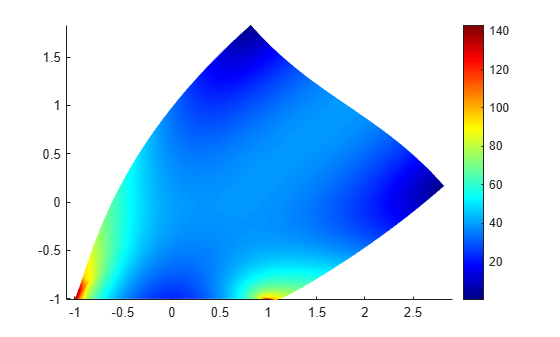Plot the deformed shape with the scale factor 500.

```pdeplot(structuralmodel, ... "XYData",structuralresults.VonMisesStress, ... "Deformation",structuralresults.Displacement, ... "DeformationScaleFactor",500,... "ColorMap","jet")```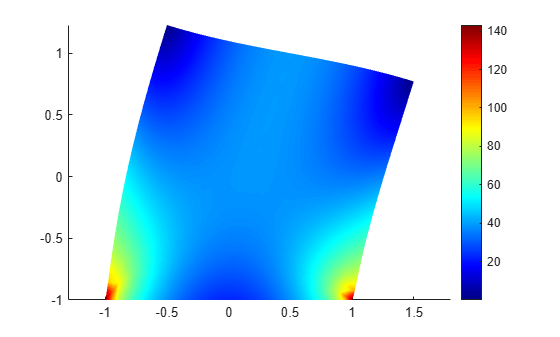Plot the deformed shape without scaling.

```pdeplot(structuralmodel,"XYData",structuralresults.VonMisesStress, ... "ColorMap","jet")```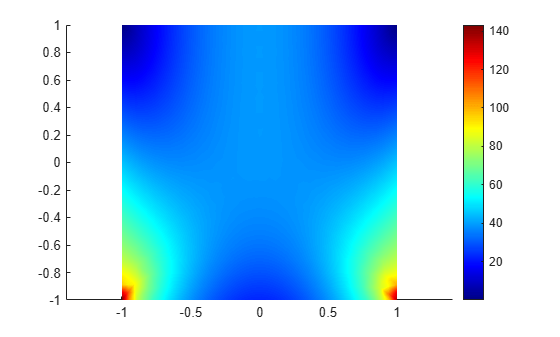Find the fundamental (lowest) mode of a 2-D cantilevered beam, assuming prevalence of the plane-stress condition.

Specify geometric and structural properties of the beam, along with a unit plane-stress thickness.

```length = 5; height = 0.1; E = 3E7; nu = 0.3; rho = 0.3/386;```

Create a modal plane-stress model, assign a geometry, and generate a mesh.

```structuralmodel = createpde("structural","modal-planestress"); gdm = [3;4;0;length;length;0;0;0;height;height]; g = decsg(gdm,'S1',('S1')'); geometryFromEdges(structuralmodel,g);```

Define a maximum element size (five elements through the beam thickness).

```hmax = height/5; msh=generateMesh(structuralmodel,"Hmax",hmax);```

Specify the structural properties and boundary constraints.

```structuralProperties(structuralmodel,"YoungsModulus",E, ... "MassDensity",rho, ... "PoissonsRatio",nu); structuralBC(structuralmodel,"Edge",4,"Constraint","fixed");```

Compute the analytical fundamental frequency (Hz) using the beam theory.

```I = height^3/12; analyticalOmega1 = 3.516*sqrt(E*I/(length^4*(rho*height)))/(2*pi)```
```analyticalOmega1 = 126.9498 ```

Specify a frequency range that includes an analytically computed frequency and solve the model.

`modalresults = solve(structuralmodel,"FrequencyRange",[0,1e6])`
```modalresults = ModalStructuralResults with properties: NaturalFrequencies: [32x1 double] ModeShapes: [1x1 FEStruct] Mesh: [1x1 FEMesh] ```

The solver finds natural frequencies and modal displacement values at nodal locations. To access these values, use `modalresults.NaturalFrequencies` and `modalresults.ModeShapes`.

`modalresults.NaturalFrequencies/(2*pi)`
```ans = 32×1 105 × 0.0013 0.0079 0.0222 0.0433 0.0711 0.0983 0.1055 0.1462 0.1930 0.2455 ⋮ ```
`modalresults.ModeShapes`
```ans = FEStruct with properties: ux: [6511x32 double] uy: [6511x32 double] Magnitude: [6511x32 double] ```

Plot the y-component of the solution for the fundamental frequency.

```pdeplot(structuralmodel,"XYData",modalresults.ModeShapes.uy(:,1)) title(['First Mode with Frequency ', ... num2str(modalresults.NaturalFrequencies(1)/(2*pi)),' Hz']) axis equal```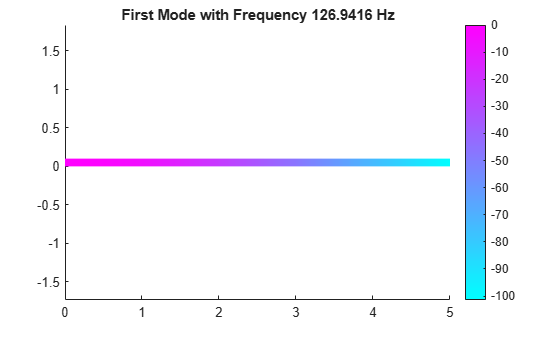Solve an electromagnetic problem and find the electric potential and field distribution for a 2-D geometry representing a plate with a hole.

Create an electromagnetic model for electrostatic analysis.

`emagmodel = createpde("electromagnetic","electrostatic");`

Import and plot the geometry representing a plate with a hole.

```importGeometry(emagmodel,"PlateHolePlanar.stl"); pdegplot(emagmodel,"EdgeLabels","on")```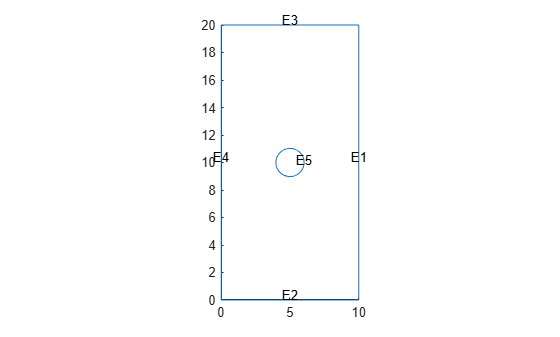Specify the vacuum permittivity value in the SI system of units.

`emagmodel.VacuumPermittivity = 8.8541878128E-12;`

Specify the relative permittivity of the material.

`electromagneticProperties(emagmodel,"RelativePermittivity",1);`

Apply the voltage boundary conditions on the edges framing the rectangle and the circle.

```electromagneticBC(emagmodel,"Voltage",0,"Edge",1:4); electromagneticBC(emagmodel,"Voltage",1000,"Edge",5);```

Specify the charge density for the entire geometry.

`electromagneticSource(emagmodel,"ChargeDensity",5E-9);`

Generate the mesh.

`generateMesh(emagmodel);`

Solve the model.

`R = solve(emagmodel)`
```R = ElectrostaticResults with properties: ElectricPotential: [1218x1 double] ElectricField: [1x1 FEStruct] ElectricFluxDensity: [1x1 FEStruct] Mesh: [1x1 FEMesh] ```

Plot the electric potential and field.

```pdeplot(emagmodel,"XYData",R.ElectricPotential, ... "FlowData",[R.ElectricField.Ex ... R.ElectricField.Ey]) axis equal```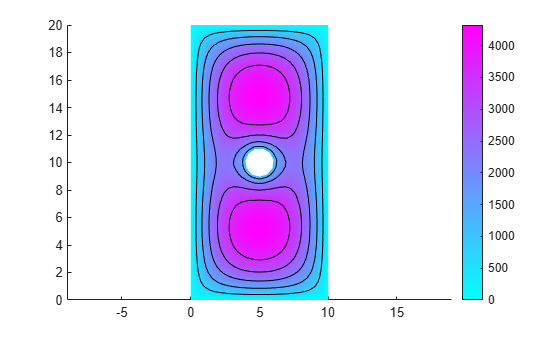Plot the `p,e,t` mesh. Display the solution using 2-D and 3-D colored plots.

Create the geometry, mesh, boundary conditions, PDE coefficients, and solution.

```[p,e,t] = initmesh('lshapeg'); u = assempde("lshapeb",p,e,t,1,0,1);```

Plot the mesh.

`pdeplot(p,e,t)`Plot the solution as a 2-D colored plot.

`pdeplot(p,e,t,"XYData",u)`Plot the solution as a 3-D colored plot.

`pdeplot(p,e,t,"XYData",u,"ZData",u)`## Input Arguments

collapse all

Model object, specified as a `PDEModel` object, `ThermalModel` object, `StructuralModel` object, or `ElectromagneticModel` object.

Example: `model = createpde(1)`

Example: ```thermalmodel = createpde("thermal","steadystate")```

Example: ```structuralmodel = createpde("structural","static-solid")```

Example: ```emagmodel = createpde("electromagnetic","magnetostatic")```

Mesh object, specified as the `Mesh` property of a `PDEModel` object or as the output of `generateMesh`.

Example: `model.Mesh`

Nodal coordinates, specified as a 2-by-NumNodes matrix. NumNodes is the number of nodes.

Element connectivity matrix in terms of the node IDs, specified as a 3-by-NumElements or 6-by-NumElements matrix. Linear meshes contain only corner nodes. For linear meshes, the connectivity matrix has three nodes per 2-D element. Quadratic meshes contain corner nodes and nodes in the middle of each edge of an element. For quadratic meshes, the connectivity matrix has six nodes per 2-D element.Mesh points, specified as a 2-by-`Np` matrix of points, where `Np` is the number of points in the mesh. For a description of the (`p`,`e`,`t`) matrices, see Mesh Data as [p,e,t] Triples.

Typically, you use the `p`, `e`, and `t` data exported from the PDE Modeler app, or generated by `initmesh` or `refinemesh`.

Example: `[p,e,t] = initmesh(gd)`

Data Types: `double`

Mesh edges, specified as a `7`-by-`Ne` matrix of edges, where `Ne` is the number of edges in the mesh. For a description of the (`p`,`e`,`t`) matrices, see Mesh Data as [p,e,t] Triples.

Typically, you use the `p`, `e`, and `t` data exported from the PDE Modeler app, or generated by `initmesh` or `refinemesh`.

Example: `[p,e,t] = initmesh(gd)`

Data Types: `double`

Mesh triangles, specified as a `4`-by-`Nt` matrix of triangles, where `Nt` is the number of triangles in the mesh. For a description of the (`p`,`e`,`t`) matrices, see Mesh Data as [p,e,t] Triples.

Typically, you use the `p`, `e`, and `t` data exported from the PDE Modeler app, or generated by `initmesh` or `refinemesh`.

Example: `[p,e,t] = initmesh(gd)`

Data Types: `double`

### Name-Value Arguments

Specify optional pairs of arguments as `Name1=Value1,...,NameN=ValueN`, where `Name` is the argument name and `Value` is the corresponding value. Name-value arguments must appear after other arguments, but the order of the pairs does not matter.

Before R2021a, use commas to separate each name and value, and enclose `Name` in quotes.

Example: `pdeplot(model,"XYData",u,"ZData",u)`

When you use a `PDEModel` object, `pdeplot(model,"XYData",u,"ZData",u)` sets surface plot coloring to the solution `u`, and sets the heights for a 3-D plot to `u`. Here `u` is a `NodalSolution` property of the PDE results returned by `solvepde` or `solvepdeeig`.

When you use a `[p,e,t]` representation, `pdeplot(p,e,t,"XYData",u,"ZData",u)` sets surface plot coloring to the solution `u` and sets the heights for a 3-D plot to the solution `u`. Here `u` is a solution returned by a legacy solver, such as `assempde`.

Tip

Specify at least one of the `FlowData` (vector field plot), `XYData` (colored surface plot), or `ZData` (3-D height plot) name-value pairs. Otherwise, `pdeplot` plots the mesh with no data.

Data Plots

collapse all

Colored surface plot data, specified as a vector. If you use a `[p,e,t]` representation, specify data for points in a vector of length `size(p,2)`, or specify data for triangles in a vector of length `size(t,2)`.

• Typically, you set `XYData` to the solution `u`. The `pdeplot` function uses `XYData` for coloring both 2-D and 3-D plots.

• `pdeplot` uses the colormap specified in the `ColorMap` name-value pair, using the style specified in the `XYStyle` name-value pair.

• When the `Contour` name-value pair is `"on"`, `pdeplot` also plots level curves of `XYData`.

• `pdeplot` plots the real part of complex data.

To plot the `k`th component of a solution to a PDE system, extract the relevant part of the solution. For example, when using a `PDEModel` object, specify:

```results = solvepde(model); u = results.NodalSolution; % each column of u has one component of u pdeplot(model,"XYData",u(:,k)) % data for column k```

When using a `[p,e,t]` representation, specify:

```np = size(p,2); % number of node points uk = reshape(u,np,[]); % each uk column has one component of u pdeplot(p,e,t,"XYData",uk(:,k)) % data for column k```

Example: `"XYData",u`

Data Types: `double`

Coloring choice, specified as one of the following values:

• `"off"` — No shading, only mesh is displayed.

• `"flat"` — Each triangle in the mesh has a uniform color.

• `"interp"` — Plot coloring is smoothly interpolated.

The coloring choice relates to the `XYData` name-value pair.

Example: `"XYStyle","flat"`

Data Types: `char` | `string`

Data for the 3-D plot heights, specified as a matrix. If you use a `[p,e,t]` representation, provide data for points in a vector of length `size(p,2)` or data for triangles in a vector of length `size(t,2)`.

• Typically, you set `ZData` to `u`, the solution. The `XYData` name-value pair sets the coloring of the 3-D plot.

• The `ZStyle` name-value pair specifies whether the plot is continuous or discontinuous.

• `pdeplot` plots the real part of complex data.

To plot the `k`th component of a solution to a PDE system, extract the relevant part of the solution. For example, when using a `PDEModel` object, specify:

```results = solvepde(model); u = results.NodalSolution; % each column of u has one component of u pdeplot(model,"XYData",u(:,k),"ZData",u(:,k)) % data for column k```

When using a `[p,e,t]` representation, specify:

```np = size(p,2); % number of node points uk = reshape(u,np,[]); % each uk column has one component of u pdeplot(p,e,t,"XYData",uk(:,k),"ZData",uk(:,k)) % data for column k```

Example: `"ZData",u`

Data Types: `double`

3-D plot style, specified as one of these values:

• `"off"` — No 3-D plot.

• `"discontinuous"` — Each triangle in the mesh has a uniform height in a 3-D plot.

• `"continuous"` — 3-D surface plot is continuous.

If you use `ZStyle` without specifying the `ZData` name-value pair, then `pdeplot` ignores `ZStyle`.

Example: `"ZStyle","discontinuous"`

Data Types: `char` | `string`

Data for the quiver plot, specified as an `M`-by-`2` matrix, where `M` is the number of mesh nodes. `FlowData` contains the x and y values of the field at the mesh points.

When you use a `PDEModel` object, set `FlowData` as follows:

```results = solvepde(model); gradx = results.XGradients; grady = results.YGradients; pdeplot(model,"FlowData",[gradx grady])```

When you use a `[p,e,t]` representation, set `FlowData` as follows:

```[gradx,grady] = pdegrad(p,t,u); % Calculate gradient pdeplot(p,e,t,"FlowData",[gradx;grady])```

When you use `ZData` to represent a 2-D PDE solution as a 3-D plot and you also include a quiver plot, the quiver plot appears in the z = 0 plane.

`pdeplot` plots the real part of complex data.

Example: `"FlowData",[ux uy]`

Data Types: `double`

Indicator to show the quiver plot, specified as `"arrow"` or `"off"`. Here, `"arrow"` displays the quiver plot specified by the `FlowData` name-value pair.

Example: `"FlowStyle","off"`

Data Types: `char` | `string`

Indicator to convert the mesh data to x-y grid before plotting, specified as `"off"` or `"on"`.

Note

This conversion can change the geometry and lessen the quality of the plot.

By default, the grid has about `sqrt(size(t,2))` elements in each direction.

Example: `"XYGrid","on"`

Data Types: `char` | `string`

Customized x-y grid, specified as a matrix `[tn;a2;a3]`. For example:

```[~,tn,a2,a3] = tri2grid(p,t,u,x,y); pdeplot(p,e,t,"XYGrid","on","GridParam",[tn;a2;a3],"XYData",u)```

For details on the grid data and its `x` and `y` arguments, see `tri2grid`. The `tri2grid` function does not work with `PDEModel` objects.

Example: `"GridParam",[tn;a2;a3]`

Data Types: `double`

Mesh Plots

collapse all

Node labels, specified as `"off"` or `"on"`.

`pdeplot` ignores `NodeLabels` when you use it with `ZData`.

Example: `"NodeLabels","on"`

Data Types: `char` | `string`

Element labels, specified as `"off"` or `"on"`.

`pdeplot` ignores `ElementLabels` when you use it with `ZData`.

Example: `"ElementLabels","on"`

Data Types: `char` | `string`

Structural Analysis Plots

collapse all

Data for plotting the deformed shape for a structural analysis model, specified as the `Displacement` property of the `StaticStructuralResults` object.

In an undeformed shape, center nodes in quadratic meshes are always added at half-distance between corners. When you plot a deformed shape, the center nodes might move away from the edge centers.

Example: `"Deformation",structuralresults.Displacement`

Scaling factor for plotting the deformed shape, specified as a real number. Use this argument with the `Deformation` name-value pair. The default value is defined internally, based on the dimensions of the geometry and the magnitude of the deformation.

Example: `"DeformationScaleFactor",100`

Data Types: `double`

Annotations and Appearance

collapse all

Indicator to include a color bar, specified as `"on"` or `"off"`. Specify `"on"` to display a bar giving the numeric values of colors in the plot. For details, see `colorbar`. The `pdeplot` function uses the colormap specified in the `ColorMap` name-value pair.

Example: `"ColorBar","off"`

Data Types: `char` | `string`

Colormap, specified as a value representing a built-in colormap, or a colormap matrix. For details, see `colormap`.

`ColorMap` must be used with the `XYData` name-value pair.

Example: `"ColorMap","jet"`

Data Types: `double` | `char` | `string`

Indicator to show the mesh, specified as `"on"` or `"off"`. Specify `"on"` to show the mesh in the plot.

Example: `"Mesh","on"`

Data Types: `char` | `string`

Title of plot, specified as a character vector or a string.

Example: `"Title","Solution Plot"`

Data Types: `char` | `string`

Surface transparency for 3-D geometry, specified as a real number from `0` through `1`. The default value `1` indicates no transparency. The value `0` indicates complete transparency.

Example: `"FaceAlpha",0.5`

Data Types: `double`

Indicator to plot level curves, specified as `"off"` or `"on"`. Specify `"on"` to plot level curves for the `XYData` data. Specify the levels with the `Levels` name-value pair.

Example: `"Contour","on"`

Data Types: `char` | `string`

Levels for contour plot, specified as a positive integer or a vector of level values.

• Positive integer — Plot `Levels` as equally spaced contours.

• Vector — Plot contours at the values in `Levels`.

To obtain a contour plot, set the `Contour` name-value pair to `"on"`.

Example: `"Levels",16`

Data Types: `double`

## Output Arguments

collapse all

Handles to graphics objects, returned as a vector.

collapse all

### Quiver Plot

A quiver plot is a plot of a vector field. It is also called a flow plot.

Arrows show the direction of the field, with the lengths of the arrows showing the relative sizes of the field strength. For details on quiver plots, see `quiver`.

## Version History

Introduced before R2006a

expand all